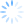# #YOLO Programming

In my last article I discussed path finding using genetic algorithms.  In that article I explained that we were using a path finding problem to have fun learning GA, but that in real life we'd want to use something far better suited to the problem, such as A*.  Since most of the code was already written for the last article, I thought I'd owe it to you to give you an example of A* in action; and since any love story is really a path finding problem between two kindred spirits, why not do it on Valentine's Day?Many of you may have learned about A* in school, as I did (back when dinosaurs roamed the Earth); it's a pretty simple algorithm used for finding the optimal path between two points, given an admissible heuristic.  It is similar to a breadth-first search algorithm in that it will end up searching all of the nodes, so if a solution exists it will find it.  To speed up the search, it uses heuristics so that only the most promising nodes are explored first.

The key components of A* are the following:

• HScore: Heuristic Cost - What is the estimated cost from the current node to the target?
• GScore: Movement Cost - What was the cost of getting to the current node?
• FScore: G + H

In our example, the heuristic cost will be the Manhattan Distance  between the current node and the target node, which makes a lot of sense for us because our maze-walker from the last article is not capable of moving in diagonals.  The movement cost will always be 1.

In English, the algorithm does the following:

• We select the node from the open list with the smallest F score.  The open list is a set of candidate nodes (nodes that are traversable [e.g. not an obstacle] and that have not been walked yet).  The first selected node is the starting node.
• We remove the current node from the open list and add it to the closed list. The closed list is a set of nodes that have already been walked.
• If the current node is the target node, we can return since the problem is now solved (yay!)
• Since we have not yet reached the target, we need to move from the current node.  We'll look at the list of the current node's neighbors and
• If the neighbor is traversable and not in the closed list
• Calculate the G Score and recalculate the F score
• If the neighbor is not in the open list, we set it's parent to the current node add it to the open list
• If the neighbor is in the open list and has a smaller new F Score than what is stored currently, update the G and F scores in the node and set the parent to current
• Loop to the beginning.  If the open list is empty then there is no path between start and target.

If you're interested in code, you can check out a c# implementation on GitHub.

So how much better is A* than our implementation using Genetic Algorithms?  Here's a solution path using the same maze as the last article (now with a geeky Valentine's Day theme).  It found the solution in milliseconds, whereas the GA took minutes!If you're interested in learning more about A*, with optimizations and tricks, check out this video series.

Happy coding and Happy Valentines Day!